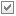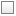# Using the Macro

Execute the CM_synthesis_and_design.lua application macro in POSTFEKO to calculate the weighted sum for the characteristic modes of interest.

1. Open antenna_plate.fek in POSTFEKO.
2. Execute the CMA_synthesis_and_design.lua application macro in POSTFEKO.
The Characteristic mode synthesis and design dialog is displayed.
3. In the Select CM data drop-down list, select antenna_plate.CharacteristicModeConfiguration1.CharacteristicModes1 and click OK.
The second Characteristic mode synthesis and design dialog is displayed.
4. In the Frequency (Hz) drop-down list, select a frequency.
5. In the Definition method drop-down list, select one of the following.
• Select Synthesis, to use the modal weighting coefficient as the weight for each mode interest.
Note: The synthesis mode requires the modal excitation coefficients to be calculated.
• Select Design, to specify the weighting coefficient manually for each mode of interest.
6. Select the modes of interest to calculate the weighted sum.
Tip: Use thebutton to select all modes and thebutton to clear all modes.
7. If Design is selected, enter the weights for the selected modes, for each mode.
• Enter a value for the real part of the complex weight Weight x:Re.
• Enter a value for the imaginary part of the complex weight Im.
8. Specify the results to calculate a weighted sum for the characteristic modes.
• Select the Include far fields check box, to calculate a weighted sum for the far field data.
• Select the Include near fields check box, to calculate a weighted sum for the near field data.
• Select the Include surface currents check box, to calculate a weighted sum for the surface current data.
• Select the Include wire currents check box, to calculate a weighted sum for the wire current data.
9. Click Calculate to start the calculation process.
The following dialogs are displayed.
10. View the results under stored data.
Note: The label convention for the synthesis definition methods are:
• Synthesis: <requestname>_SM1__M20.
• Design: <requestname>_DM1__M20.
An example using the synthesis definition method, summing modes 1 to 20 at 830 MHz for the antenna plate example. The results for the far field and currents are shown. The full solution (MoM) is compared with the synthesis method.